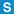# class ZipCommon

Library: Zip
Package: Zip

## Description

Common enums used in the Zip project

## Member Summary

Member Functions: isValidPath

## Enumerations

CL_NORMAL = 0

CL_MAXIMUM = 1

CL_FAST = 2

CL_SUPERFAST = 3

### CompressionMethod

CM_STORE = 0

CM_SHRUNK = 1

CM_FACTOR1 = 2

CM_FACTOR2 = 3

CM_FACTOR3 = 4

CM_FACTOR4 = 5

CM_IMPLODE = 6

CM_TOKENIZE = 7

CM_DEFLATE = 8

CM_UNUSED = 11

CM_AUTO = 255

automatically select DM_DEFLATE or CM_STORE based on file type (extension)

FT_BINARY = 0

FT_ASCII = 1

HS_FAT = 0

HS_AMIGA = 1

HS_VMS = 2

HS_UNIX = 3

HS_VM_CMS = 4

HS_ATARI = 5

HS_HPFS = 6

HS_MACINTOSH = 7

HS_ZSYSTEM = 8

HS_CP_M = 9

HS_TOPS20 = 10

HS_NTFS = 11

HS_SMS_QDOS = 12

HS_ACORN = 13

HS_VFAT = 14

HS_MVS = 15

HS_BEOS = 16

HS_TANDEM = 17

HS_UNUSED = 18

## Member Functions

### isValidPathstatic bool isValidPath(
const std::string & path
);

Checks whether the given path is valid (does not contain ".." path segments).

## Variables

### ZIP64_EXTRA_IDstatic const Poco::UInt16 ZIP64_EXTRA_ID = 0x1;

### ZIP64_MAGICstatic const Poco::UInt32 ZIP64_MAGIC = 0xFFFFFFFF;

### ZIP64_MAGIC_SHORTstatic const Poco::UInt16 ZIP64_MAGIC_SHORT = 0xFFFF;